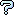All about flooble | fun stuff | Get a free chatterbox | Free JavaScript | Avatarsperplexus dot infoAnd equality for all.... (Posted on 2007-12-11)Find the largest number, a single string of only digits - without radix or exponent, that can be equated to a mathematical expression that uses no variables and where each and every digit, 0 to 9, is used but once in the equation. Provide the equation.
Mathematical symbols and functions are permitted, yet limit each to less than the arbitrary number of ten.
If limited to digits 0 to 5, a possible answer, for example, could be:
5!/(4 - 3) = 120.

 Submitted by Dej Mar Rating: 2.5000 (2 votes) Solution: (Hide) This submission was more a challenge than a puzzle, though puzzling it may have been.Under the requirements proposed, ideally the equation would be of the form F(x) = n, where F(x) was the combination of various math functions using the single smallest digit, i.e., 0 to seed the function and n equalling the largest number possible with the remaining digits, i.e., 987654321. Using various math functions the result might possibly be attained. For instance, examine the following equation: |_cosh(|_(cosh(]tan(0!)[)_|)_|! - 4 - 1 = 3628795such that |_x_| is the floor function and ]x[ is the ceiling function.As can be seen, with a single 0 a much larger number was acquired. When I had submitted the challenge, I had limited myself to only a few functions. As such, the largest candidate I've found was: p8# + 6\$ * 1 = 9734250 p8# (or 8#, the primorial of 8; 1st definition) = 9699690; 6\$ (the superfactorial of 6) = 34560 As to the largest number for the digits 0 to 5, one candidate might be:(5!! - 4)# = 23105!! (the double factorial of 5) = 15; 11# (the primorial of 11; 2nd definition) = 2310Comments: ( You must be logged in to post comments.)
 Subject Author Dateimproved ... a little Ady TZIDON 2007-12-15 12:29:03re(2): 6 digits (3 x10^6) Leming 2007-12-12 22:49:57re: 6 digits (3 x10^6) Ady TZIDON 2007-12-12 18:41:51re: 6 digits (3 x10^6) Ady TZIDON 2007-12-12 18:38:116 digits (3 x10^6) Leming 2007-12-12 18:18:15re(2): three MORE Ady TZIDON 2007-12-12 17:16:53re: three MORE Leming 2007-12-12 16:06:47three MORE Ady TZIDON 2007-12-12 15:44:02ONE OF MANY STUPID SOLUTIONS Ady TZIDON 2007-12-12 15:34:35It's just that easy! Jyqm 2007-12-11 19:55:11A little less, but no operations on the right side. Leming 2007-12-11 16:33:50re: another - not quite right - answer Dej Mar 2007-12-11 12:44:02another - not quite right - answer Leming 2007-12-11 11:27:00So close - but off by one number. Leming 2007-12-11 11:18:13Please log in:

 Search: Search body:
Forums (0)